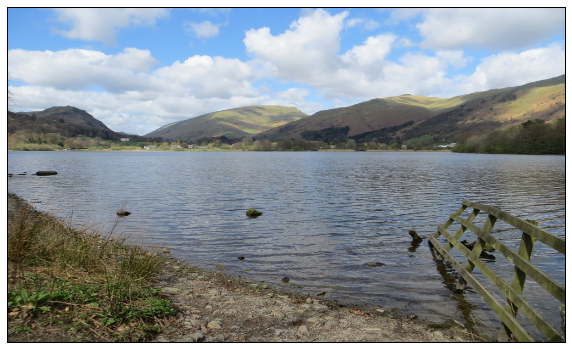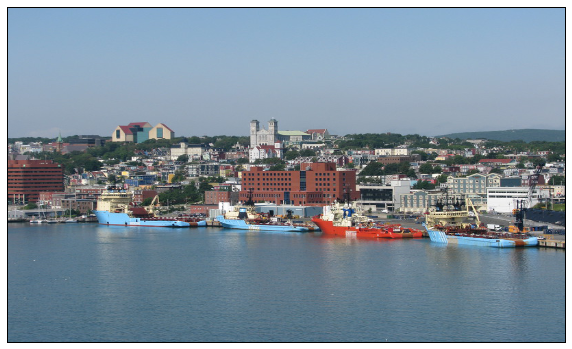# The Artificial Artist

Inspired by cubism and various projects using genetic algorithms to paint the Mona Lisa here a method for teaching your computer to be an artist!

I bring you the artificial artist!

The idea is to use regression to "learn" what an image looks like and then draw the learned image. By choosing the parameters of the regressor carefully you can achieve some interesting visual effects.

If all this artsy talk is too much for you think of this as a way to compress an image. You could store the weights of the regressor instead of the whole image.

First some standard imports of things we will need later:

In :
%matplotlib inline

In :
from base64 import b64encode
from tempfile import NamedTemporaryFile

import numpy as np
import scipy
import matplotlib.pyplot as plt
from matplotlib import animation
from IPython.display import HTML
from sklearn.ensemble import RandomForestRegressor as RFR
from skimage.color import rgb2lab,lab2rgb
from skimage.transform import resize,rescale

from JSAnimation import IPython_display


## Pretty pictures¶

First, some pictures to work with. The first one was taken during a trip to the Lake district in north England. The second one is a shot of the harbour in St. John's in Newfoundland.

In :
lakes = imread('http://betatim.github.io/images/artificial-arts/lakes.jpg')
f,ax = plt.subplots(figsize=(10,6))
ax.xaxis.set_ticks([]); ax.yaxis.set_ticks([])
ax.imshow(lakes, aspect='auto')

Out:
<matplotlib.image.AxesImage at 0x11e47a850>In :
newfie = imread('http://betatim.github.io/images/artificial-arts/newfie.jpg')
f,ax = plt.subplots(figsize=(10,6))
ax.xaxis.set_ticks([]); ax.yaxis.set_ticks([])
ax.imshow(newfie, aspect='auto')

Out:
<matplotlib.image.AxesImage at 0x122aadf50>## The Artificial Artist: a new Kind of Instagram Filter¶

The artificial artist will be based on a decision tree regressor using the $(x,y)$ coordinates of each pixel in the image as features and the RGB values as the target. Once the tree has been trained we ask it to make a prediction for every pixel in the image, this is our image with the "filter" applied. All this is taken care of in the simple_cubist function. Once you see the first filtered image you will understand why it is called simple_cubist.

We also define a compare function which takes care of displaying several images next to each other or compiling them into an animation. This makes it easy to see what we just did.

In :
def simple_cubist(img):
w,h = img.shape[:2]
img = rgb2lab(img)

xx,yy = np.meshgrid(np.arange(w), np.arange(h))
X = np.column_stack((xx.reshape(-1,1), yy.reshape(-1,1)))

Y = img.reshape(-1,3, order='F')

min_samples = int(round(0.001 * len(X)))

model = RFR(n_estimators=1,
n_jobs=6,
max_depth=None,
min_samples_leaf=min_samples,
random_state=43252,
)
model.fit(X, Y)
art_img = model.predict(X)
art_img = art_img.reshape(w,h,3, order='F')

return lab2rgb(art_img)

def compare(*imgs, **kwds):
"""Draw several images at once for easy comparison"""
animate = kwds.get("animate", False)

if animate:
fig, ax = plt.subplots(figsize=(8,5))
ax.xaxis.set_ticks([])
ax.yaxis.set_ticks([])
anim = animation.FuncAnimation(fig,
lambda x: ax.imshow(imgs[x%len(imgs)],
aspect='auto'),
frames=len(imgs),
interval=1000)

fig.tight_layout()
return anim

else:
figsize = plt.figaspect(len(imgs)) * 1.5
fig, axes = plt.subplots(nrows=len(imgs), figsize=figsize)
for a in axes:
a.xaxis.set_ticks([])
a.yaxis.set_ticks([])

for ax,img in zip(axes,imgs):
ax.imshow(img)

fig.tight_layout()

# Take the picture of the Lake District and apply our
# simple cubist filter to it
simple_lakes = simple_cubist(lakes)
compare(lakes, simple_lakes, animate=True)

Out:

Once Loop Reflect